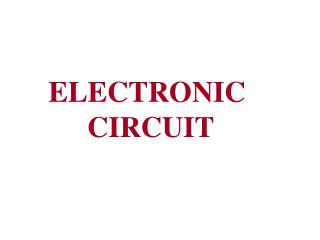DownloadDownload PresentationELECTRONIC CIRCUIT

# ELECTRONIC CIRCUIT

Télécharger la présentation## ELECTRONIC CIRCUIT

- - - - - - - - - - - - - - - - - - - - - - - - - - - E N D - - - - - - - - - - - - - - - - - - - - - - - - - - -
##### Presentation Transcript

1. ELECTRONIC CIRCUIT

2. Practical Voltage Source

3. Practical Current Source

4. Circuit Topology Fundamental

5. Definition of a branch

6. Definitions of node and supernode

7. (a) A circuit containing three nodes and five branches. (b) Node 1 is redrawn to look like two nodes; it is still one node.

8. Definition of a loop Definition of a mesh

9. Series Circuits and Kirchhoff’s Voltage Law

10. Kirchhoff’s Voltage Law The sum of the component voltages in a series circuit must equal the source voltage1840 – German Physicist, Gustav Kirchhoff Actual wording – The algebraic sum of the voltages around a closed loop is zero The following equation takes polarity into account Voltage Relationships: Kirchhoff’s Voltage Law

11. Example: VS = +10V, V1 = +2V, V2 = +8V Kirchhoff’s Voltage Law,

12. Series Circuit – a circuit that contains only one current path Series Circuit Characteristics

13. (a) Series combination of N resistors. (b) Electrically equivalent circuit.

14. Series Circuit Characteristics • Total Series Resistance where RT = the total circuit resistance Rn = the highest-numbered resistor in the circuit

15. Current Characteristics – the current at any point in a series circuit must equal the current at every other point in the circuit Series Circuit Characteristics Insert Figure 4.5

16. Voltage Characteristics Series Circuit Characteristics where VS = the source (or total) voltage Vn = the voltage across the highest numbered resistor in the circuit

17. (a) Series connected voltage sources can be replaced by a single source. (b) Parallel current sources can be replaced by a single source.

18. Examples of circuits with multiple sources, some of which are “illegal” as they violate Kirchhoff’s laws.

19. Power Characteristics Series Circuit Characteristics where PS = the source (or total) voltage Pn = the power that is dissipated across the highest numbered resistor in the circuit

20. Series Circuit Characteristics Insert Figure 4.10

21. Voltage References - Circuits have a point that serves as the 0 V reference (ground) Voltage References Insert Figure 4.12

22. The Voltage Divider Relationship Voltage Divider – often used to analyze a series circuit Voltage Divider where Rn = the resistor of interest Vn = the voltage drop across Rn (where n is the component number)

23. We may find v2 by applying KVL and Ohm’s law: so Thus, An illustration of voltage division. or For a string of N series resistors, we may write:

24. Source Resistance: A Practical Consideration Ideal Voltage Source – maintains a constant output voltage regardless of the resistance of its load Real Voltage Source – internal resistance causes a decrease in load resistance results in a decrease in the source voltage

25. Source Resistance: A Practical Consideration (Continued) Insert Figure 4.20

26. maximum power transfer from a voltage source to its load occurs when the load resistance is equal to the source resistance Maximum Power Transfer Theorem

27. Series-Aiding Voltage Sources – the total voltage equals the sum of the voltages Series-Opposing Voltage Sources – the total voltage equals the difference of the voltages Series-Connected Voltage Sources

28. Earth Ground Versus Chassis Ground Insert Figure 4.28

29. Parallel Circuitsand Kirchholf’s Current Law

30. Kirchhoff’s Current Law: The algebraic sum of the currents entering and leaving a point must equal zero In other words, the total current leaving a point must equal the total current entering that point i i 2 1 i 3 Current Relationships: Kirchhoff’s Current Law

31. Parallel Circuit – a circuit that provides more than one current path between any two points Parallel Circuit Characteristics Insert Figure 5.1

32. Current Characteristics Parallel Circuit Characteristics where In = the current through the highest-numbered branch in the circuit

33. Voltage and Current Values Voltage across each component is equal Current through each branch is determined by the source voltage and the resistance of the branch. Parallel Circuit Characteristics

34. Resistance Characteristics – the total circuit resistance is always lower than any of the branch resistance values Parallel Circuit Characteristics Insert Figure 5.5

35. Power Characteristics Total Power – sum of the power dissipation values for the individual components The lower value of the branch resistance, the higher percentage of the total power it dissipates (opposite that of series circuits) Parallel Circuit Characteristics

36. Parallel Circuit Characteristics Insert Figure 5.6

37. Beginning with a simple KCL equation, or Thus, A special case worth remembering is (a) A circuit with N resistors in parallel. (b) Equivalent circuit. Example:

38. Calculating Total Resistance: The Product-Over-Sum Method Parallel Resistance Relationships

39. a source that is designed to provide an output current value that remains relatively constant over a wide range of load resistance values Current Sources Insert Figure 5.12

40. Current Dividers – the source current is divided among the branches Current Dividers

41. The current flowing through R2 is or An illustration of current division. For a parallel combination of N resistors, the current through Rk is

42. The Effects of Source Resistance Ideal Current Source – constant current and infinite internal resistance Real Current Source – current varies for a change in load resistance and internal resistance is not infinite Internal resistance is usually much greater than the load resistance Practical Current Sources:

43. Series-Parallel Circuits

44. Connecting Series Circuits in Parallel Series-Parallel Circuits Insert Figure 6.3

45. Connecting Parallel Circuits in Series Series-Parallel Circuits Insert Figure 6.5

46. Analyzing Series-Parallel Circuits